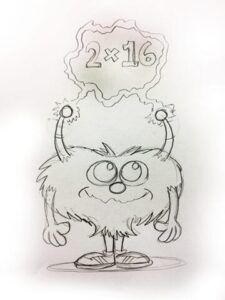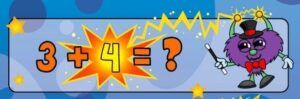Select Page

# Math Mights Mania: Introducing Abracus

Apr 27, 2018

This week, allow me to introduce Abracus!

The second of our Math Mights featured in the Math Mights 8-in-1 Flip Chart: Addition Strategies, Abracus holds a magic wand and uses the strategy of compensation. Many times people confuse the strategies of decomposing/composing and compensation because, in some instances, you might compensate a problem similarly to how you would decompose and compose with D.C.

### What is Compensation?This strategy of compensation, represented by Abracus, is usually first taught in 1st grade, after students learn decomposing/composing, and it is usually known as doubles +/- 1 or double +/- 2 concept.

Abracus was developed to be a bit of a sneaky or a magical character. While he’s in Mathville, he can kind of transform or compensate a number with his magical wand to make it a friendlier number that he wanted it to be. Then would also have the magic to transform that number back to the original quantity that was being added.

If Abracus were to approach the problem of 29 + 15, he would zap the 29 into a 30 by rounding up and holding on to the extra 1 in his head. Then, he is left with 30 + 15, which is much more simple to compute. But the answer wouldn’t be 45, because Abracua still has to zap the 30 back into 29. So, after he does that, he is left with 44 as his answer.

Lots of kids can do this kind of problem mentally. Developmentally, we first teach students the strategy of decomposing and composing. However, when numbers need to be rounded because they’re close, are close to another decade or another friendly number, kids often can go up a little bit or down a little bit depending on where they are and how they compensate.

Compensation allows children to work with higher numbers, and even allows them to build up to compensating both numbers, helps them with doubles +1, doubles +2 and doubles -1 and doubles -2.

### Compensation in Action

An interesting thing about compensation is that you can compensate just one number at the beginning before you begin and change it back later – we usually call this Give One Get One (GOGO). For example, in the problem 28 + 22, we can change the number 28 by adding 2 to it to get 30. I could then compute the two numbers together (30 + 22) in the problem to get a total (52). Finally, I would subtract 2 from the final number to get the correct number (50).

When students are using this strategy, I want to make sure that students (especially younger learners) make note of what they’re compensating. If they are changing a 28 to a 30, make a note that they added 2 so they don’t forget to take off the 2.

Most math books teach students to compensate both numbers at the same time. If I had 28 + 22, I could easily add 2 to 28 and subtract 2 from 22, which would give me a nice problem of 30 + 20. This is double compensating – adding or giving 2 and taking away 2 at the same time.

### Beginning StrategiesIn the flip chart of addition strategies, Abracus shows different examples of how you can use the compensation strategy with different kinds of numbers. In 1st grade, he might encounter a problem like 3 + 4 (which is a doubles +1). I know 3 + 3 is 6, but Abracus zaps the number 4 by subtracting a 1, and so I have to add it back in at the end after I get a total to get 7 as my answer to the problem.

Most kids don’t really know what you mean when you say “doubles +1” or “doubles -1” A) because they’re probably still learning their doubles, but B) because it sounds very complicated mathematically if they can’t see a picture for it. You want kids to be able to use a manipulative of sorts, like a double-ten frame, where you could put 6 double-sided counters in the top row and five on the bottom to show kids that the “double” happens when you take one counter off the top row to make the 6 a 5. Then you can actually see 5 and 5. But, you took it off, so you have to add it back on. You can also demonstrate the same concept with a Rekenrek or a Counting Buddy but you want kids to visualize the value of the doubles are when a certain number is taken away. You could also build an example with 7 on the top of the 10-frame and 5 on the bottom.  You’d want the kids to remove the two counters to show that you’re taking two away and you can see the 5+5 (doubles), but then you want to add two back on to get 12.

As kids start to get the hang of it, you could move compensation up to larger numbers, like 15+16. A child might know what 15+15 is because they can benchmark to that easily to get to 30. Technically, Abracus zapped 16 to make it 15, so, after they add 15+15 to get 30. Finally, they’ll add the 1 to get 31.

The same idea applies when you work with numbers in quantities of 25 where kids might think of coins. You might have 25 + 27, and a kid might choose to have Abracus zap 2 off of 27 to get 25+25, which is 50. And then later, Abracus remembers to add back in the 2 he zapped earlier to get the total of 52. Kids can play with this idea and then start to learn to compensate both numbers.On the advanced side of the flip chart, Abracus shows us how he does compensation with decimals. If I had 1.9 + 2.1, it would be very simple to add .1  on to 1.9 to make it 2, and then on the other number, I could subtract .1 to make it 2. That makes this problem very easy to add because you can compensate both of the numbers.

You can also compensate in larger amount. If a student wanted to subtract 9, they could, but they might want to roll up one more depending what numbers they’re working with.

This character shows a nice connection in the vertical alignment of the flip chart from the students understanding the early strategy of doubles +1 but being able to do it with higher numbers. They also could do this with money as well, making sure that it’s always an even trade.Compensation for subtraction is much different that it is for addition, so we want to make sure kids understand this character of Abracus very well before we move on. Typically, we think of addition and multiplication together as having similar characteristics, and subtraction and division together. As a result, we’ll use a different character in the subtraction poster to help kids understand the idea of families (even within Mathville!). Compensation for subtraction is represented by a different character because it is a very different strategy because it involves actually shifting the number line. When we launch our subtraction poster, you’ll meet Mini and Subbie (Minuend and Subtrahend), who are actually pictured at the top of our Addition Strategies poster.